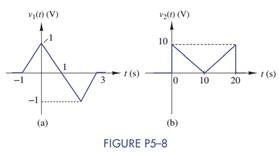Create an Account

Home / Questions / Express each of the waveforms in Figure P5–8 as a sum of singularity functions.

Express each of the waveforms in Figure P5–8 as a sum of singularity functions.

Express each of the waveforms in Figure P5–8 as a sum of singularity functions.Jul 28 2020 View more View LessSubscribe To Get Solution